# Java Applet | Implementing Flood Fill algorithm

• Last Updated : 11 May, 2021

Flood Fill Algorithm is to replace a certain closed or a similarly colored field with a specified color. The use of the FloodFill algorithm can be seen in paints and other games such as minesweeper.
In this article, FloodFill is used for a connected area by a specified color, in Java Applet by using the FloodFill algorithm.
There are two approaches that can be used:

1. Recursive approach (limited usage as it crashes for a larger area)
2. Using queue (more reliable)

Examples:

1. For image 1: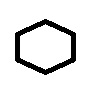• Output (floodfilled at position 35, 35):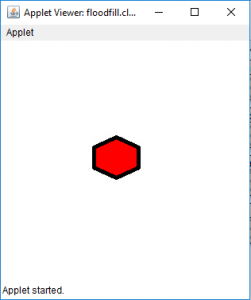•
• Output (floodfilled at position 1, 1):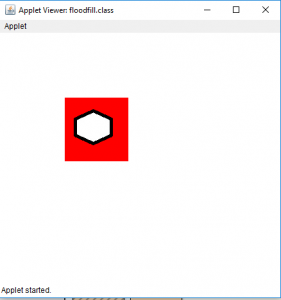1. For image 2: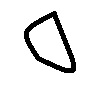• Output(floodfilled at position 35, 35) :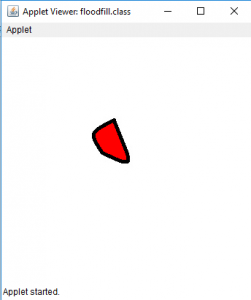• Output (floodfilled at position 1, 1):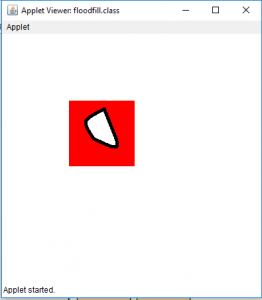• />
1. For image 3: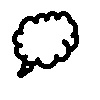• Output(floodfilled at position 35, 35) :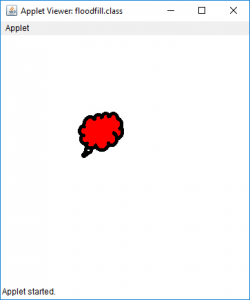• Output (floodfilled at position 1, 1):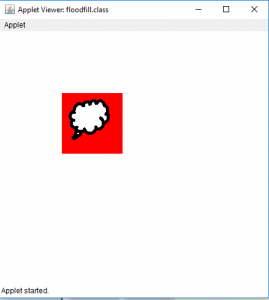Program 1: To implement floodfill algorithm in Java Applet using recursion:
Note: To run the program, use an offline IDE such as Netbeans, Eclipse, etc. Please download the input images and put them along with the class file. Otherwise, the program might yield an “Can’t read the input file” error.

## Java

 `// Java Program to implement floodfill algorithm` `// in Java Applet(using recursion)` `import` `java.awt.*;` `import` `javax.swing.*;` `import` `java.awt.image.*;` `import` `java.io.*;` `import` `javax.imageio.ImageIO;`   `public` `class` `floodfill ``extends` `JApplet {`   `    ``public` `void` `init()` `    ``{` `    ``}`   `    ``// paint function` `    ``public` `void` `paint(Graphics g)` `    ``{` `        ``BufferedImage i = ``null``;` `        ``try` `{` `            ``// Input the image to be used for FloodFill` `            ``// The output is shown for 3 images` `            ``// image1, image2 and image2` `            ``i = ImageIO.read(``new` `File(``"image1.jpg"``));`   `            ``// floodfill with color red at point 35, 35` `            ``// get color of image at 35, 35` `            ``Color c = ``new` `Color(i.getRGB(``35``, ``35``));` `            ``flood(i, g, ``35``, ``35``, c, Color.red);`   `            ``// draw the image after floodfill` `            ``g.drawImage(i, ``100``, ``100``, ``this``);` `        ``}` `        ``catch` `(Exception e) {` `            ``JOptionPane.showMessageDialog(``this``, e.getMessage());` `        ``}`   `        ``// draw the image after floodfill` `        ``g.drawImage(i, ``100``, ``100``, ``this``);` `    ``}`   `    ``// function to floodfill the image` `    ``public` `void` `flood(BufferedImage i,` `                      ``Graphics g,` `                      ``int` `x,` `                      ``int` `y,` `                      ``Color c,` `                      ``Color c1)` `    ``{` `        ``if` `(x >= ``1` `&& y >= ``1` `            ``&& x < i.getWidth()` `            ``&& y < i.getHeight()) {` `            ``// find the color at point x, y` `            ``Color c2 = ``new` `Color(i.getRGB(x, y));`   `            ``// if there is no boundary (the color is almost` `            ``// same as the color of the point where` `            ``// floodfill is to be applied` `            ``if` `(Math.abs(c2.getGreen() - c.getGreen()) < ``30` `                ``&& Math.abs(c2.getRed() - c.getRed()) < ``30` `                ``&& Math.abs(c2.getBlue() - c.getBlue()) < ``30``) {` `                ``// change the color of the pixel of image` `                ``i.setRGB(x, y, c1.getRGB());`   `                ``g.drawImage(i, ``100``, ``100``, ``this``);`   `                ``// floodfill in all possible directions` `                ``flood(i, g, x, y + ``1``, c, c1);` `                ``flood(i, g, x + ``1``, y, c, c1);` `                ``flood(i, g, x - ``1``, y, c, c1);` `                ``flood(i, g, x, y - ``1``, c, c1);` `            ``}` `        ``}` `    ``}` `}`

Output:

• for image 1:
Input:• Output :•
• for image 2:
Input:• Output :•
• for image 3:
Input:• Output :• Note: If a larger area is flood filled (at coordinate 1, 1) using the recursive approach, then recursive algorithm might get crashed.
Example:

```floodfill the larger side of image
Input:````Output:`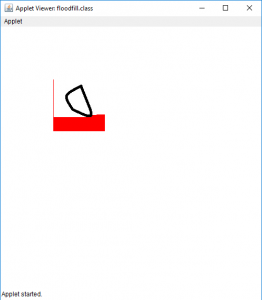```Explanation:
Since the area to be covered is very large,
therefore only some part is covered by the algorithm,
and after that the program gets crashed.```
• Program 2: To implement floodfill algorithm in Java Applet using queue:
Note: To run the program, use an offline IDE such as Netbeans, Eclipse, etc. Please download the input images and put them along with the class file. Otherwise, the program might yield an “Can’t read the input file” error.

## Java

 `// Java Program to implement floodfill algorithm` `// in Java Applet(using queue)` `import` `java.awt.*;` `import` `javax.swing.*;` `import` `java.awt.image.*;` `import` `java.io.*;` `import` `javax.imageio.ImageIO;`   `public` `class` `floodfill ``extends` `JApplet {`   `    ``public` `void` `init()` `    ``{` `    ``}`   `    ``// paint function` `    ``public` `void` `paint(Graphics g)` `    ``{` `        ``BufferedImage i = ``null``;` `        ``try` `{` `            ``// Input the image to be used for FloodFill` `            ``// The output is shown for 3 images` `            ``// image1, image2 and image2` `            ``i = ImageIO.read(``new` `File(``"image1.jpg"``));`   `            ``// floodfill with color red at point 1, 1` `            ``// get color of image at 1, 1` `            ``// if 35, 35 point is floodfilled it will floodfill` `            ``// the smaller area` `            ``Color c = ``new` `Color(i.getRGB(``1``, ``1``));` `            ``flood(i, g, ``1``, ``1``, c, Color.red);`   `            ``// draw the image after floodfill` `            ``g.drawImage(i, ``100``, ``100``, ``this``);` `        ``}` `        ``catch` `(Exception e) {` `            ``JOptionPane.showMessageDialog(``this``, e.getMessage());` `        ``}`   `        ``// draw the image after floodfill` `        ``g.drawImage(i, ``100``, ``100``, ``this``);` `    ``}`   `    ``// function to floodfill the image using queue` `    ``public` `void` `flood(BufferedImage i,` `                      ``Graphics g,` `                      ``int` `x1,` `                      ``int` `y1,` `                      ``Color c,` `                      ``Color c1)` `    ``{` `        ``// create a stack using array` `        ``int` `stx[] = ``new` `int``[``100000``];` `        ``int` `sty[] = ``new` `int``[``100000``], f, r, x, y;`   `        ``// create a front and rear` `        ``f = r = ``0``;`   `        ``// initialize them` `        ``stx[``0``] = x1;` `        ``sty[``0``] = y1;`   `        ``// while front is greater than rear` `        ``while` `(f >= r) {` `            ``// pop element out` `            ``x = stx[r];` `            ``y = sty[r++];` `            ``if` `(x >= ``1` `&& y >= ``1` `                ``&& x < i.getWidth()` `                ``&& y < i.getHeight()) {` `                ``// find the color at point x, y` `                ``Color c2 = ``new` `Color(i.getRGB(x, y));`   `                ``// if there is no boundary (the color is almost` `                ``// same as the color of the point where` `                ``// floodfill is to be applied`   `                ``if` `(Math.abs(c2.getGreen() - c.getGreen()) < ``30` `                    ``&& Math.abs(c2.getRed() - c.getRed()) < ``30` `                    ``&& Math.abs(c2.getBlue() - c.getBlue()) < ``30``) {`   `                    ``// change the color of the pixel of image` `                    ``i.setRGB(x, y, c1.getRGB());`   `                    ``g.drawImage(i, ``100``, ``100``, ``this``);`   `                    ``// floodfill in all possible directions` `                    ``// store them in queue` `                    ``stx[f] = x;` `                    ``sty[f++] = y + ``1``;` `                    ``stx[f] = x;` `                    ``sty[f++] = y - ``1``;` `                    ``stx[f] = x + ``1``;` `                    ``sty[f++] = y;` `                    ``stx[f] = x - ``1``;` `                    ``sty[f++] = y;` `                ``}` `            ``}` `        ``}` `    ``}` `}`

• Output:
• For image 1:
Input:• Output (floodfilled at position 35, 35):• Output (floodfilled at position 1, 1):•
• For image 2:
Input:• Output(floodfilled at position 35, 35) :• Output (floodfilled at position 1, 1):•
• For image 3:
Input:• Output(floodfilled at position 35, 35) :• Output (floodfilled at position 1, 1):My Personal Notes arrow_drop_up
Recommended Articles
Page :